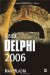Nested if-then Statements

If you need even more control over application execution, you can write nested if-then statements. Nesting if-then statements enables you to define very specific conditions under which your code can successfully execute. A very simple example of a nested if-then statement is an application that tells the user if a number is odd or even, but only if the user enters an integer value larger than 0.

Listing 3-4: A nested if-then statementprogram Project1; {\$APPTYPE CONSOLE} uses   SysUtils; var   Num: Integer; begin   Write('Enter a number: ');   ReadLn(Num);   if Num > 0 then     if Num mod 2 = 0  then       WriteLn('An even number.')     else       WriteLn('An odd number.')   else     WriteLn('The number has to be larger than zero!');   ReadLn; end.This simple application illustrates a somewhat hard-to-read nested if-then statement that uses the mod operator to see if the number is odd or even. If not for the indentations in the code, these two else reserved words could cause a lot of trouble for an unwary programmer. A much better (read: recommended) solution is to write the inner if-then statement in a block:

if Num > 0 then begin   if Num mod 2 = 0  then     WriteLn('An even number.')   else     WriteLn('An odd number.'); end else   WriteLn('The number has to be larger than zero!');Inside Delphi 2006 (Wordware Delphi Developers Library)
ISBN: 1598220039
EAN: 2147483647
Year: 2004
Pages: 212